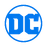## FANDOM

109,577 PagesThis page contains a listing of all known appearances of Borb Borbb (New Earth).
If you find an Issue this Character appears in that is not shown here, please edit that issue adding "Borb Borbb (New Earth)" as a Cast member.

All items (43)

D
•Darkstars Vol 1 11
•Darkstars Vol 1 12
•DCU: Trinity Vol 1 2
G
•Green Lantern Vol 3 45
L
•L.E.G.I.O.N. Vol 1 30
•L.E.G.I.O.N. Vol 1 31
•L.E.G.I.O.N. Vol 1 32
•L.E.G.I.O.N. Vol 1 34
•L.E.G.I.O.N. Vol 1 35
•L.E.G.I.O.N. Vol 1 36
•L.E.G.I.O.N. Vol 1 37
•L.E.G.I.O.N. Vol 1 38
•L.E.G.I.O.N. Vol 1 39
•L.E.G.I.O.N. Vol 1 40
•L.E.G.I.O.N. Vol 1 44
•L.E.G.I.O.N. Vol 1 45
•L.E.G.I.O.N. Vol 1 50
•L.E.G.I.O.N. Vol 1 52
•L.E.G.I.O.N. Vol 1 53
•L.E.G.I.O.N. Vol 1 54
•L.E.G.I.O.N. Vol 1 57
•L.E.G.I.O.N. Vol 1 58
•L.E.G.I.O.N. Vol 1 59
•L.E.G.I.O.N. Vol 1 62
•L.E.G.I.O.N. Vol 1 64
•L.E.G.I.O.N. Vol 1 65
•L.E.G.I.O.N. Vol 1 66
•L.E.G.I.O.N. Vol 1 70
R
•R.E.B.E.L.S. Vol 1 0
•R.E.B.E.L.S. Vol 1 1
•R.E.B.E.L.S. Vol 1 2
•R.E.B.E.L.S. Vol 1 3
•R.E.B.E.L.S. Vol 1 4
•R.E.B.E.L.S. Vol 1 5
•R.E.B.E.L.S. Vol 1 6
•R.E.B.E.L.S. Vol 1 7
•R.E.B.E.L.S. Vol 1 8
•R.E.B.E.L.S. Vol 1 9
•R.E.B.E.L.S. Vol 1 10
•R.E.B.E.L.S. Vol 1 12
•R.E.B.E.L.S. Vol 1 13
•R.E.B.E.L.S. Vol 1 14
•R.E.B.E.L.S. Vol 1 15
Community content is available under CC-BY-SA unless otherwise noted.